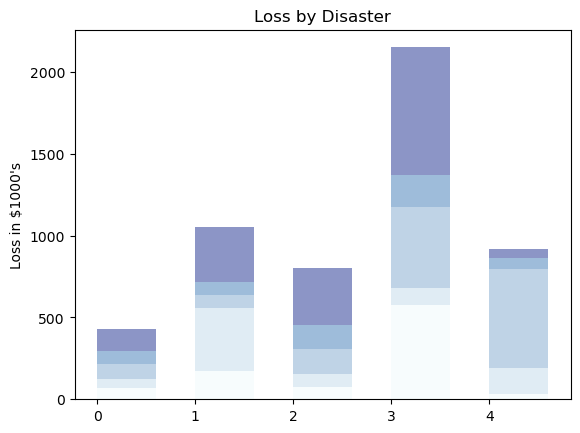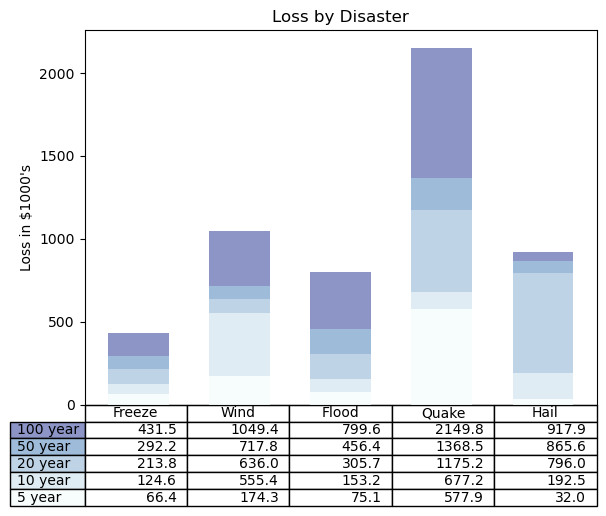## Libraries

First, you need to install the following librairies:

• matplotlib is used for plot creating the charts
• pandas is used to put the data into a dataframe and custom the table
• `numpy` for data generation
``````import pandas as pd
import matplotlib.pyplot as plt
import numpy as np``````

## Dataset

Our dataset consists of values on weather such as Freeze or Hail. We store our data in a pandas dataframe.

The "rows" column:

• for each value of x in the tuple, it creates a string like "100 year", "50 year", "20 year", and so on.
``````data = {'Freeze': [ 66386, 174296,  75131, 577908,  32015],
'Wind': [ 58230, 381139,  78045,  99308, 160454],
'Flood': [ 89135,  80552, 152558, 497981, 603535],
'Quake': [ 78415,  81858, 150656, 193263,  69638],
'Hail': [139361, 331509, 343164, 781380,  52269],
'rows': ['%d year' % x for x in (100, 50, 20, 10, 5)]}
df = pd.DataFrame(data)``````

## Create the barplot

The following code creates a stacked barplot, with a gradient color for subgroups in each bar.

• `index` specifies the positions of the bars on the x-axis
• `bar_width` determines the width of the bars
• `y_offset` is used to keep track of the vertical position of each category's bars on the chart

Then, we loop through the data and creates the stacked bar chart:

• For each row of data, it adds a set of bars to the chart
• Each bar represents a category's loss, and they are stacked on top of each other
• Color of the bar is defined with our `colors` variabme
• The heights of the bars are determined by the values in the data
``````values = np.arange(0, 2500, 500)
value_increment = 1000

# Get some pastel shades for the colors
colors = plt.cm.BuPu(np.linspace(0, 0.5, len(df)))
n_rows = len(df)

index = np.arange(len(df.columns)-1) + 0.3
bar_width = 0.6

# Initialize the vertical-offset for the stacked bar chart.
y_offset = np.zeros(len(df.columns)-1)

# Plot bars and create text labels for the table
cell_text = []
for row in range(n_rows):
plt.bar(index, df.iloc[:, row], bar_width, bottom=y_offset, color=colors[row])
y_offset = y_offset + df.iloc[:, row]

plt.ylabel(f"Loss in \${value_increment}'s")
plt.yticks(values * value_increment, ['%d' % val for val in values])
plt.title('Loss by Disaster')

# Display the chart
plt.show()``````## Add table on the chart

Using the same code as before, we add the following elements:

• It adjusts the layout with `plt.subplots_adjust(left=0.1, bottom=0.1)` to make room for the table at the bottom of the chart
• It adds a table at the bottom of the chart using `plt.table()`. This table includes the text labels, row labels (from the 'rows' column in the DataFrame), and column labels (from the DataFrame's columns)
``````values = np.arange(0, 2500, 500)
value_increment = 1000

# Get some pastel shades for the colors
colors = plt.cm.BuPu(np.linspace(0, 0.5, len(df)))
n_rows = len(df)

index = np.arange(len(df.columns)-1) + 0.3
bar_width = 0.6

# Initialize the vertical-offset for the stacked bar chart.
y_offset = np.zeros(len(df.columns)-1)

# Plot bars and create text labels for the table
cell_text = []
for row in range(n_rows):
plt.bar(index, df.iloc[:, row], bar_width, bottom=y_offset, color=colors[row])
y_offset = y_offset + df.iloc[:, row]
cell_text.append(['%1.1f' % (x / 1000.0) for x in y_offset])

# Reverse colors and text labels to display the last value at the top.
colors = colors[::-1]
cell_text.reverse()

# Add a table at the bottom of the axes
the_table = plt.table(cellText=cell_text,
rowLabels=df['rows'],
rowColours=colors,
colLabels=df.columns,
loc='bottom')

# Adjust layout to make room for the table:

plt.ylabel(f"Loss in \${value_increment}'s")
plt.yticks(values * value_increment, ['%d' % val for val in values])
plt.xticks([])
plt.title('Loss by Disaster')

# Dislay the chart
plt.show()``````## Going further

This post explains how to create a bar chart with a table underneath using matplotlib.

For more examples of how to create or customize your bar plots, see the barplot section. You may also be interested in how to create a grouped barplot.

## Contact & Edit

👋 This document is a work by Yan Holtz. You can contribute on github, send me a feedback on twitter or subscribe to the newsletter to know when new examples are published! 🔥